# RS Aggarwal Solutions for Class 6 Maths Chapter 11 Line Segment, Ray and Line

RS Aggarwal Solutions for Class 6 Chapter 11 Line Segment, Ray and Line are provided here. By practising RS Aggarwal Solutions for Class 6 Maths Chapter 11, students not only gain confidence in Mathematics but also score high marks in examinations. Students can refer RS Aggarwal Solutions by meticulously practising the textbook questions, which help them to clear their concepts and doubts pertaining to the chapter.

Experts at BYJU’S have solved RS Aggarwal textbook questions for Class 6 Chapter 11 available in PDF Format for students to follow. Download PDF of Class 6 RS Aggarwal Solutions of Chapter 11 from the below respective link.

## Download PDF of RS Aggarwal Solutions for Class 6 Chapter 11 Line Segment, Ray and Line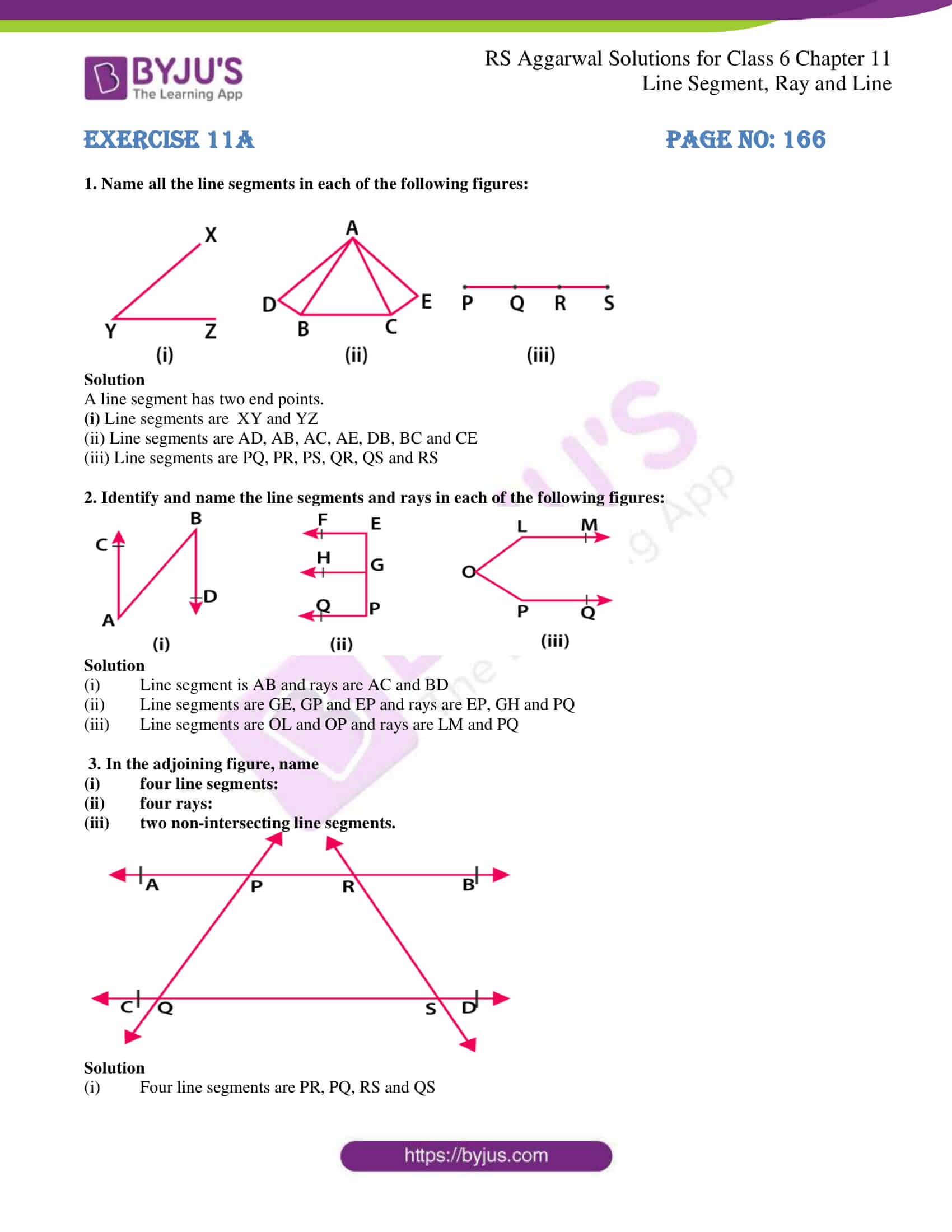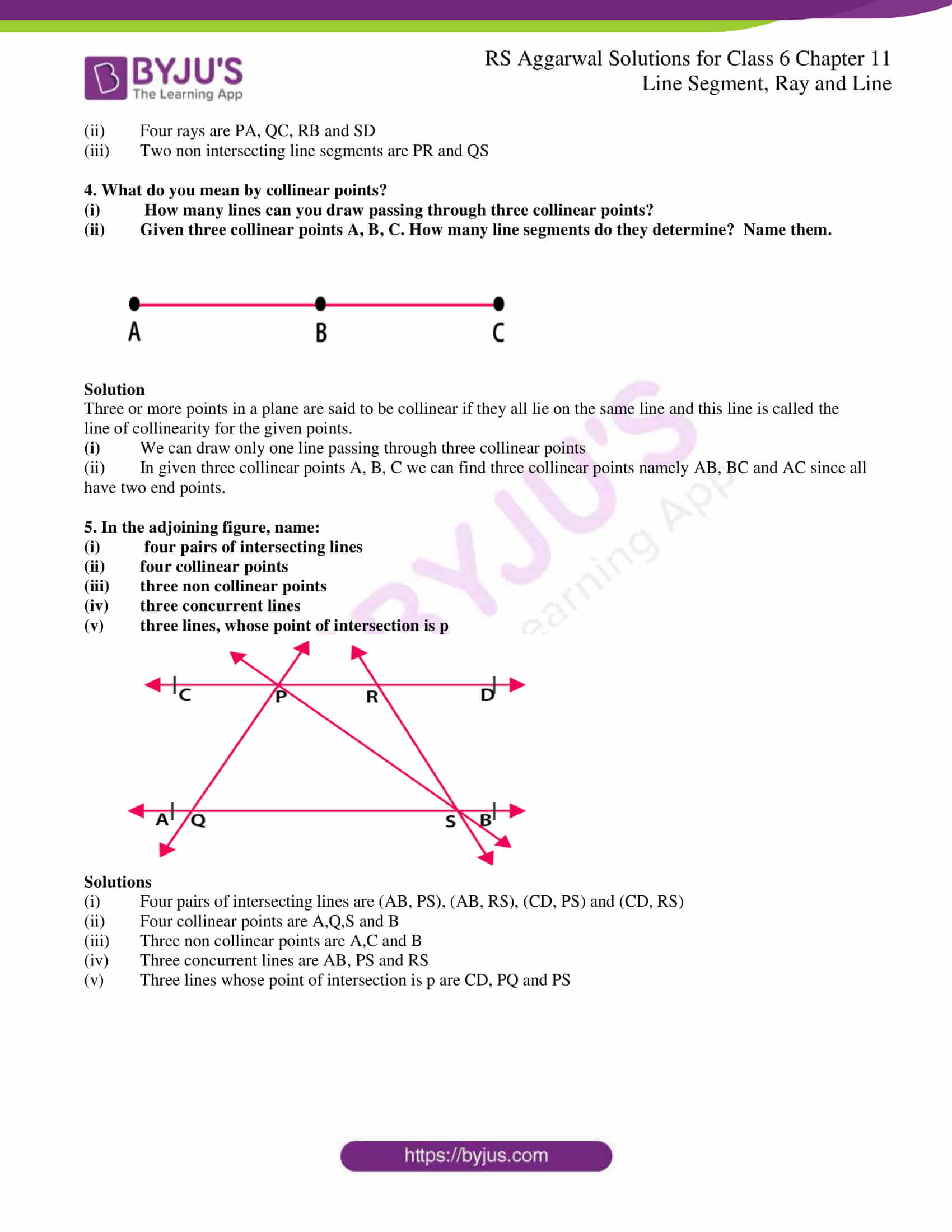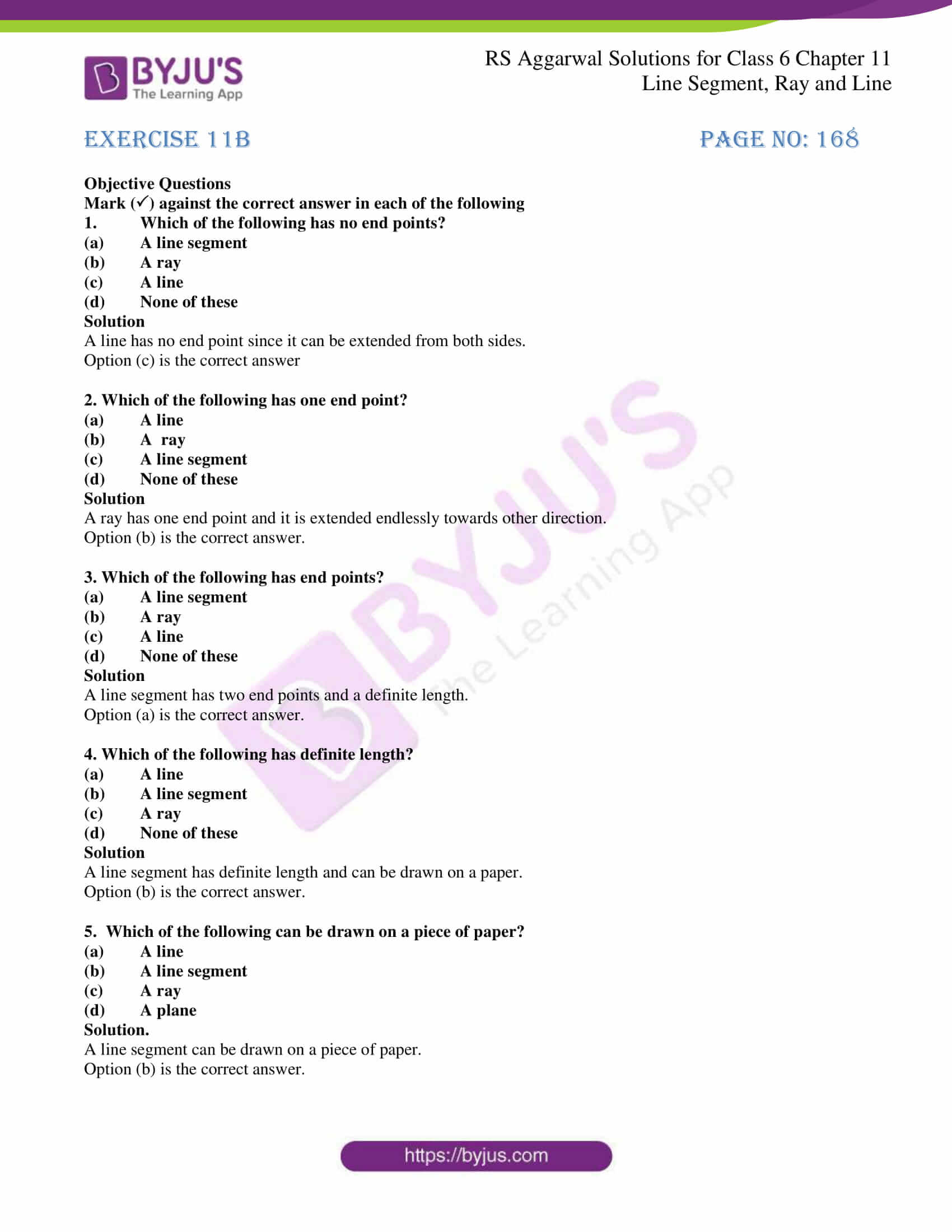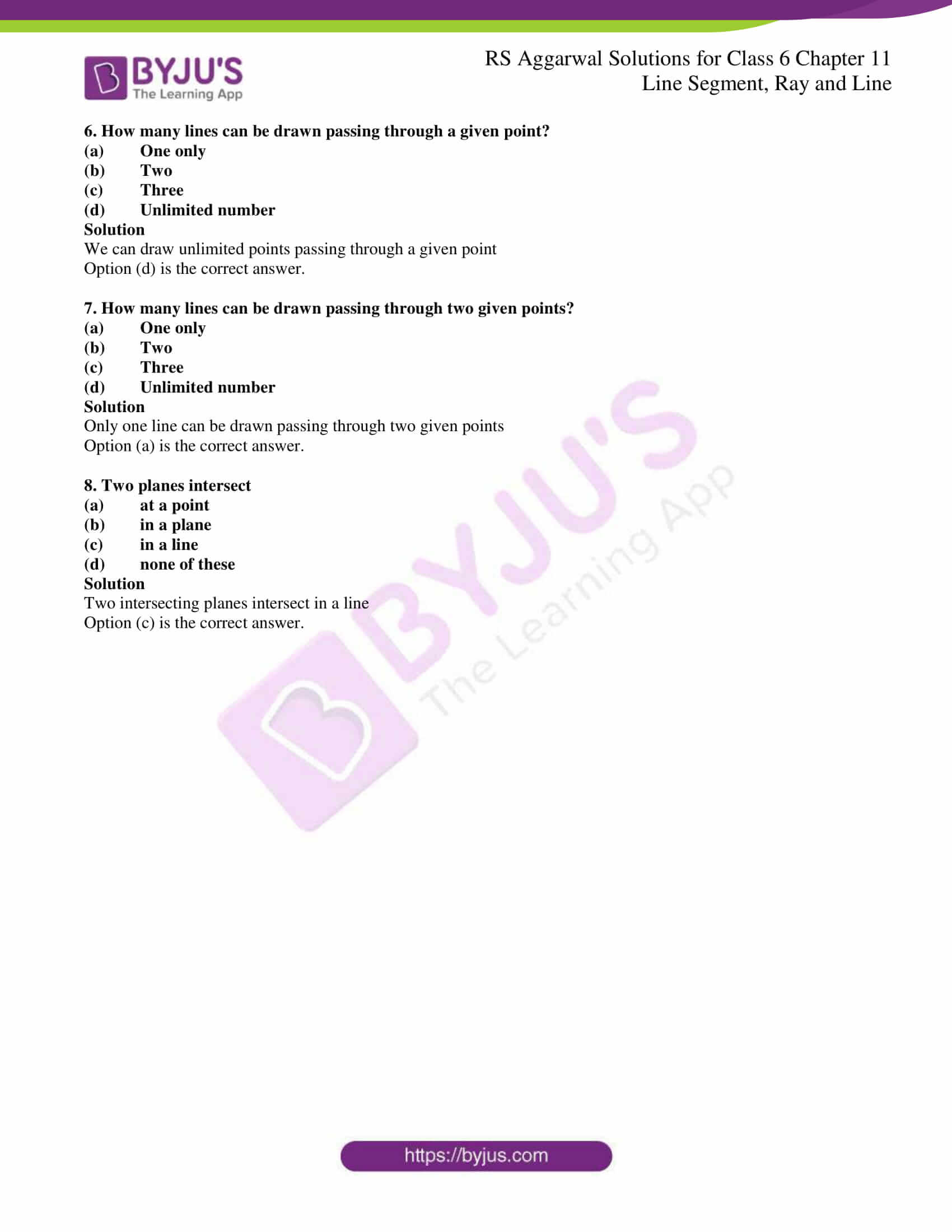### Access answers to chapter 11- Line Segment, Ray and Line

Exercise 11A PAGE NO: 166

1. Name all the line segments in each of the following figures: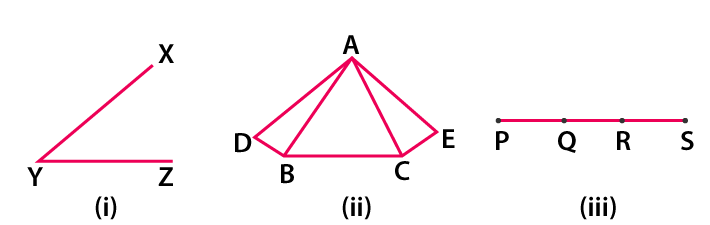Solution

A line segment has two end points.

(i) Line segments are XY and YZ

(ii) Line segments are AD, AB, AC, AE, DB, BC and CE

(iii) Line segments are PQ, PR, PS, QR, QS and RS

2. Identify and name the line segments and rays in each of the following figures: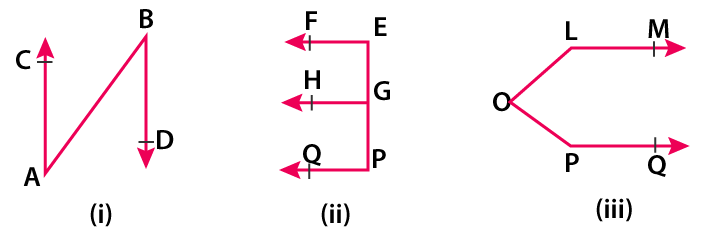Solution

(i) Line segment is AB and rays are AC and BD

(ii) Line segments are GE, GP and EP and rays are EP, GH and PQ

(iii) Line segments are OL and OP and rays are LM and PQ

3. In the adjoining figure, name

(i) four line segments:

(ii) four rays:

(iii) two non-intersecting line segments.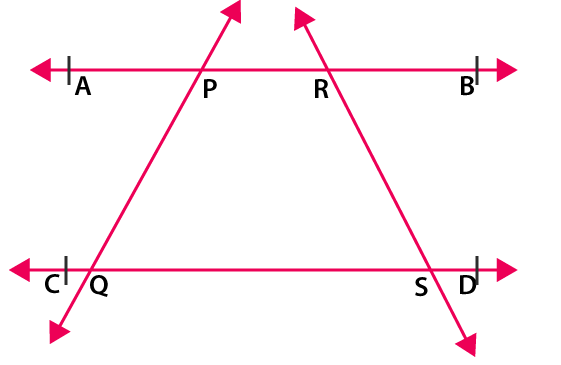Solution

(i) Four line segments are PR, PQ, RS and QS

(ii) Four rays are PA, QC, RB and SD

(iii) Two non intersecting line segments are PR and QS

4. What do you mean by collinear points?

(i) How many lines can you draw passing through three collinear points?

(ii) Given three collinear points A, B, C. How many line segments do they determine? Name them.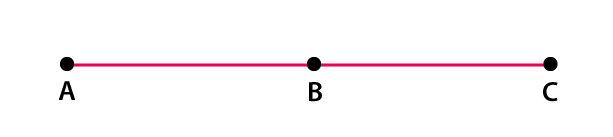Solution

Three or more points in a plane are said to be collinear if they all lie on the same line and this line is called the line of collinearity for the given points.

(i) We can draw only one line passing through three collinear points

(ii) In given three collinear points A, B, C we can find three collinear points namely AB, BC and AC since all have two end points.

5. In the adjoining figure, name:

(i) four pairs of intersecting lines

(ii) four collinear points

(iii) three non collinear points

(iv) three concurrent lines

(v) three lines, whose point of intersection is p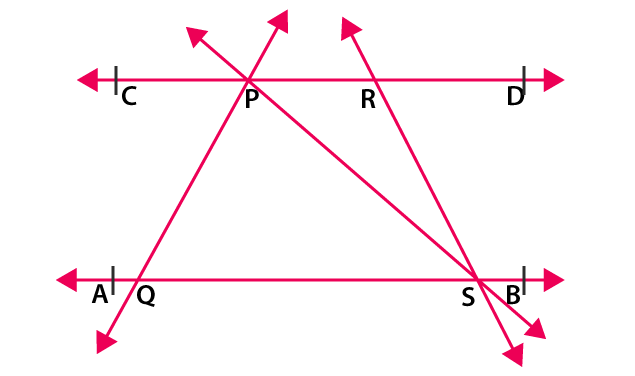Solutions

(i) Four pairs of intersecting lines are (AB, PS), (AB, RS), (CD, PS) and (CD, RS)

(ii) Four collinear points are A,Q,S and B

(iii) Three non collinear points are A,C and B

(iv) Three concurrent lines are AB, PS and RS

(v) Three lines whose point of intersection is p are CD, PQ and PS

Exercise 11B PAGE NO: 168

Objective Questions

Mark () against the correct answer in each of the following

1. Which of the following has no end points?

(a) A line segment

(b) A ray

(c) A line

(d) None of these

Solution

A line has no end point since it can be extended from both sides.

Option (c) is the correct answer

2. Which of the following has one end point?

(a) A line

(b) A ray

(c) A line segment

(d) None of these

Solution

A ray has one end point and it is extended endlessly towards other direction.

Option (b) is the correct answer.

3. Which of the following has end points?

(a) A line segment

(b) A ray

(c) A line

(d) None of these

Solution

A line segment has two end points and a definite length.

Option (a) is the correct answer.

4. Which of the following has definite length?

(a) A line

(b) A line segment

(c) A ray

(d) None of these

Solution

A line segment has definite length and can be drawn on a paper.

Option (b) is the correct answer.

5. Which of the following can be drawn on a piece of paper?

(a) A line

(b) A line segment

(c) A ray

(d) A plane

Solution.

A line segment can be drawn on a piece of paper.

Option (b) is the correct answer.

6. How many lines can be drawn passing through a given point?

(a) One only

(b) Two

(c) Three

(d) Unlimited number

Solution

We can draw unlimited points passing through a given point

Option (d) is the correct answer.

7. How many lines can be drawn passing through two given points?

(a) One only

(b) Two

(c) Three

(d) Unlimited number

Solution

Only one line can be drawn passing through two given points

Option (a) is the correct answer.

8. Two planes intersect

(a) at a point

(b) in a plane

(c) in a line

(d) none of these

Solution

Two intersecting planes intersect in a line

Option (c) is the correct answer.

### RS Aggarwal Solutions for Class 6 Chapter 11 Line Segment, Ray and Line

Chapter 11 – Line Segment, Ray and Line consists of 2 exercises. Each and every question of RS Aggarwal Solutions has been solved in depth. Let’s have a look at the topics which are discussed in this chapter

• Plane
• Point
• Line Segment
• Ray
• Line

Also, access RS Aggarwal Solutions for 6 Chapter 11 Exercises

Exercise 11A

Exercise 11B

### Chapter Brief of RS Aggarwal Solutions for Class 6 Maths Chapter 11 – Line Segment, Ray and Line

Let A and B be two points on a plane. Then, the straight path from A to B is called the line segment AB. A line segment extended endlessly in one direction is called a ray. A line segment extended endlessly on both sides is called a line. Pen or pencil is an example of a line segment, Sun’s ray is an example of ray and two persons standing in a row is an example of line.